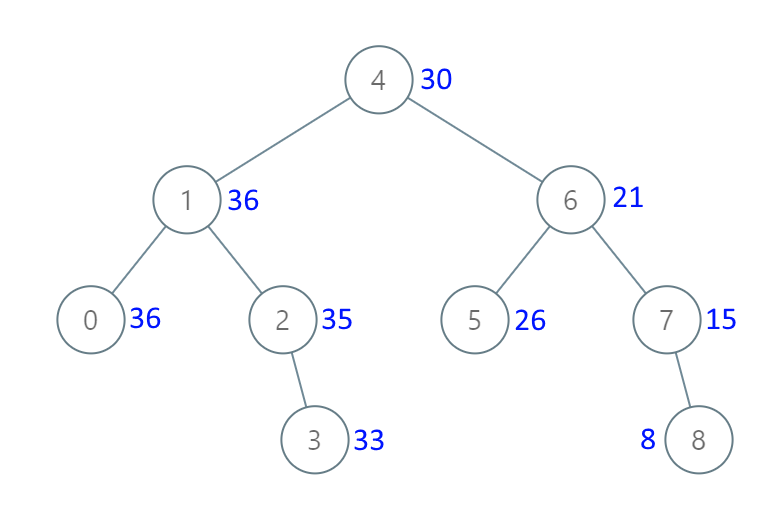# LeetCode Weekly Contest 135

https://leetcode.com/contest/weekly-contest-135

## Valid Boomerang

A boomerang is a set of 3 points that are all distinct and not in a straight line.

Given a list of three points in the plane, return whether these points are a boomerang.

Example 1:

Example 2:

Note:

1. points.length == 3
2. points[i].length == 2
3. 0 <= points[i][j] <= 100

## Binary Search Tree to Greater Sum Tree

Given the root of a binary search tree with distinct values, modify it so that every node has a new value equal to the sum of the values of the original tree that are greater than or equal to node.val.

As a reminder, a binary search tree is a tree that satisfies these constraints:

• The left subtree of a node contains only nodes with keys less than the node’s key.
• The right subtree of a node contains only nodes with keys greater than the node’s key.
• Both the left and right subtrees must also be binary search trees.

Example 1:Note:

1. The number of nodes in the tree is between 1 and 100.
2. Each node will have value between 0 and 100.
3. The given tree is a binary search tree.

## Minimum Score Triangulation of Polygon

Given N, consider a convex N-sided polygon with vertices labelled A, A[i], ..., A[N-1] in clockwise order.

Suppose you triangulate the polygon into N-2 triangles. For each triangle, the value of that triangle is the product of the labels of the vertices, and the total score of the triangulation is the sum of these values over all N-2 triangles in the triangulation.

Return the smallest possible total score that you can achieve with some triangulation of the polygon.

Example 1:

Example 2:Example 3:

Note:

1. 3 <= A.length <= 50
2. 1 <= A[i] <= 100

dp[i][j](i<j)用来表示从A[i]A[j]顺序构造的多边形划分为三角形后最高的得分，可以找一个i<k<jA[k]先构造以A[i],A[j],A[k]为顶点的三角形，那么有dp[i][j]可以是所有i<k<jk中使得dp[i][k]+dp[k][j]+A[i]*A[j]*A[k]最大的。从底向上进行动态规划时，逐步增大多边形的顶点数，即j-i+1，当顶点数为n时找到整个问题的解。

## Moving Stones Until Consecutive II

On an infinite number line, the position of the i-th stone is given by stones[i]. Call a stone an endpoint stone if it has the smallest or largest position.

Each turn, you pick up an endpoint stone and move it to an unoccupied position so that it is no longer an endpoint stone.

In particular, if the stones are at say, stones = [1,2,5], you cannot move the endpoint stone at position 5, since moving it to any position (such as 0, or 3) will still keep that stone as an endpoint stone.

The game ends when you cannot make any more moves, ie. the stones are in consecutive positions.

When the game ends, what is the minimum and maximum number of moves that you could have made? Return the answer as an length 2 array: answer = [minimum_moves, maximum_moves]

Example 1:

Example 2:

Example 3:

Note:

1. 3 <= stones.length <= 10^4
2. 1 <= stones[i] <= 10^9
3. stones[i] have distinct values.